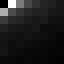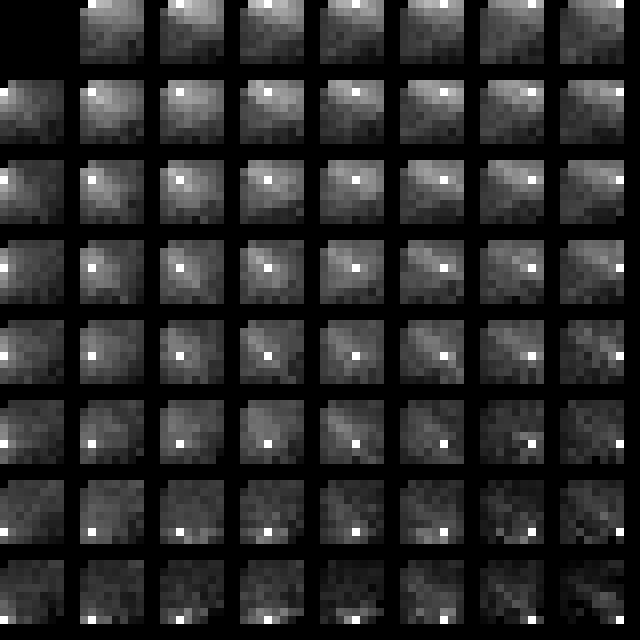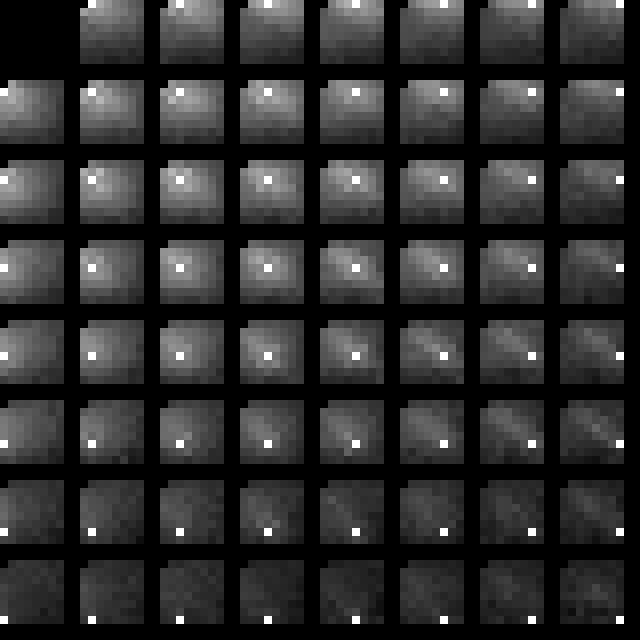# cbloom rants

## 5/26/2009

### 05-26-09 - Some Study of DCT coefficients

First of all : The number of non-zero values in the lower-diagonal area of the 8x8 block after quantization to a reasonable/typical value.

(36 of the 64 values are considered to be "lower diagonal" in the bottom right area) The actual number :

```
avg : 3.18 :
0 : 37.01
1 : 16.35
2 :  9.37
3 :  6.48
4 :  5.04
5 :  3.88
6 :  3.22
7 :  3.02
8 :  2.49
9 :  2.34
10 :  2.09
...

```
The interesting thing about this is that it has a very flat tail, much flatter than you might expect. For example, if the probability of a given coefficient being zero or nonzero was an indepedent random event, the distribution would be binomial; it peaks flatter and is then much faster to zero :
```
avg : 3.18 :
0 : 13.867587
1 : 28.162718
2 : 27.802496
3 : 17.775123
4 : 8.272518
5 : 2.986681
6 : 0.870503
7 : 0.210458
8 : 0.043037
9 : 0.007553
10 : 0.001150
...

```
What this tells us is the probability of a given coefficient being zero is highly *not* idependent. They are strongly correlated - the more values that are on, the more likely it is that the next will be on. In fact we see that if there are 6 values on, it's almost equally likely there are 7, etc. , that is : P(n)/P(n-1) goes toward 1.0 as n gets larger.

Also, amusingly the first two ratios P(1)/P(0) and P(2)/P(1) are both very close to 0.5 in every image I'm tried (in 0.4 to 0.6 generally). What this means is it wouldn't be too awful just to code the # of values on with unary, at least for the first bit (you could use something like an Elias Gamma code which uses unary at first then adds more raw bits).

Now for pretty pictures. Everyone has seen graphics like this, showing the L2 energy of each coefficient in the DCT : (none of these pictures include the DC because it's weird and different)This shows the percentage of the time the value is exactly zero :Now for some more interesting stuff. This shows the percent of correlation to the block above the current one : (to the north) :Note in particular the strong correlation of the first row.

The next one is the correlation to the block to the left (west) :Finally the fun one. This one shows the correlation of each coefficient to the other 63 coefficients in the same block :The self-correlation is 100% which makes it a white pixel obviously. Black means 0% correlation. This is absolute-value correlation in all cases (no signs). There are a lot of patterns that should be pretty obvious to the eye. Beware a bit in over-modeling on these patterns because they do change a bit from image to image, but the general trend stays the same.

And another one from a different image :This one's from Lena. A few things I think are particularly interesting - in the upper left area, which is where most of the important energy is, the correlation is most strong diagonally. That is, you see these "X" shape patterns where the center pixel is correlated mainly to it's diagonal neighbors, not the one's directly adjacent to it.

Dave Moore said...

I will win that argument by inexorable momentum. Victory will be mine!

won3d said...

How exactly is DC weird? Just the way JPEG encodes it (DPCM?) , or it just intrinsically behaves differently from the AC coefficients?

cbloom said...

"or it just intrinsically behaves differently from the AC coefficients?"

this.

For one thing you'd have to subtract off the global mean of the whole image or it would be scaled up by a huge bias.

If you delta DC from the neighboring DC's it acts more like the other coefficients, but then we're getting into details that I didn't want to involve in this test.

ryg said...

I assume the grayscale values are in linear scale, i.e. 50% gray = a correlation with magnitude of 0.5?

In any case, this is really interesting. The DCT is really obviously very far off from the optimal decorrelating transform for these images. It'd be really interesting to see how these images look for prediction residuals when doing H264-style coding.

cbloom said...

" I assume the grayscale values are in linear scale, i.e. 50% gray = a correlation with magnitude of 0.5?"

It's linear scale and actually gamma corrected, so apparent 50% brightness = correlation 0.5 (not pixel value 128).

Yeah, I agree it's somewhat shocking when you see it visually just how much correlation there is. These results are all pretty well known, but it clarifies things mentally to see the pictures.

Anonymous said...

aren't things like the number of zeroes going to depend heavily on the choice of quantizations? which are you doing here?

also, on the PCA blog post, the same issue crossed my mind: if you found the optimal transformation to some arbitrary basis, how would you quantize in that basis?

cbloom said...

"aren't things like the number of zeroes going to depend heavily on the choice of quantizations? which are you doing here?"

I'm always doing uniform quantization here BTW.

Changing quantizer absolutely changes the # of zeros, but it shouldn't affect whether a value being zero is an independent event or not.

My comment about the typical cases is based on my belief that this type of coder should only be used in the 0.25 - 1.0 bpp range.

"also, on the PCA blog post, the same issue crossed my mind: if you found the optimal transformation to some arbitrary basis, how would you quantize in that basis?"

Well you're giving up any attempts at perceptual quantization at that point. Uniform quantization in any orthonormal basis is equivalent in an R-D sense.

There is the issue that when you quantize you wind up seeing the quantization shapes. How that turns out exactly in human perceptual error is hard to say (though personally I find the claims that DCT are good in this sense to be quite dubious).